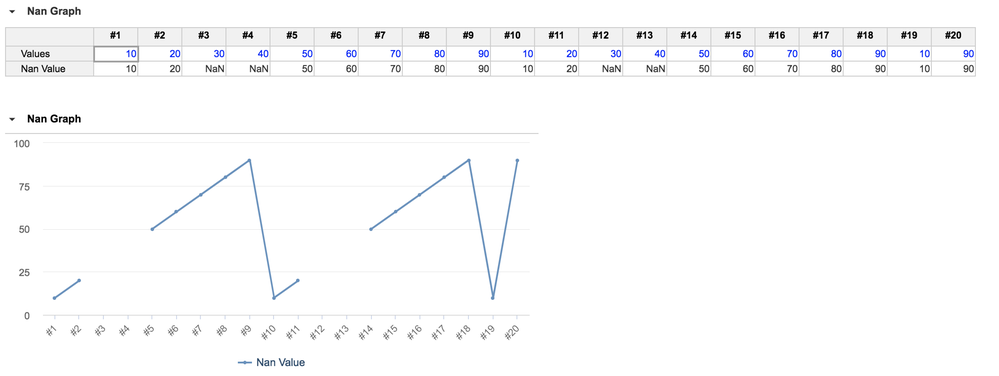# Chart creation - Omitting Null valuesHi Team,

i would like to create a chart wherein the zero values need not be plotted on a graph.In other words, I would like to create a chart excluding the null / zero values,. Please provide some guidance on the same. Existing chart screenshot is attached herewith for your ready reference.

Thanks and regards,

Aishwarya

Tagged:

•Hi Aishwarya,

To create a NaN, you need to write Divide(0,0).

Inserting NaN in the module, the following graph is derived.I prefer having 0 in my graph, because it's reflecting the true value.

But if you're still looking for a graph that bypasses 0, and connects only values, i think there's 2 choices:

1. Create a module that filters out zero, create a line chart out of the non zero values. Then at the bottom of the line chart, create a module with all values (including the zero), and use conditional formatting to highlight these zeroes, so that users can still tell when the zeroes happen.

2. The other option is to mathematically derive a value to replace the zero, so that we don't get what we see above in the NaN graph, eg if Jan = 1, Feb = 0, Mar = 5; then derive 3 for Feb so that there's a smooth line connecting Jan to Mar. However this approach has 2 problems, i.e. first of all, it sends the wrong message because i'm seeing Feb = 3 in the graph, but the module is saying 0. Secondly it's not easy to derive these values, especially when there could be more than 1 zero between values, eg Jan = 1, Feb = 0, Mar = 0, Apr = 0, May = 5.

Thanks,

LipChean

•Hi Aishwarya,

You can create a filter that only shows list members with values, then create a Saved View.

You can then create a graph out of this Saved View, and the graph should reflect accordingly, i.e. show list members with values in the graph.

Please note that this method only works with custom list, and not with Standard Time.

Thanks,

LipChean

•Thank you for the solution, we shall try it out and provide feedback!

•Hi LipChean,

I have tried your solution . Filtering the zero values is also resulting in hiding of the concerned timeperiods on the x axis. I would like to know if there is a solution wherein the x axis would display all the time periods irrespective of the quantities against them; only the chart plot needs to be blank for zero values.

Thanks and regards,

Aishwarya

•I wonder how not-a-number (NaN) is treated by the chart. If it omits them then you just need map zero to NaN. You can create a NaN using DIVIDE with zero as the second argument.
•Hi Aishwarya,

What does 'only the chart plot needs to be blank for zero values' mean?

Thanks,

LipChean

•Thank you , I shall check the same the revert to you if it works

•Hi LipChean,

I mean the chartline should be truncated at points where values are zero ( though their corresponding time periods would still remain plotted on x axis). When we filter the values, the corresponding time periods also get hidden. We would still want such time periods also to be displayed on the x axis.

Thanks and regards,

Aishwarya

•Hi Aishwarya,

Do you mean for points where the value = 0, you still want to show the time, but not the value = 0?

For example, assuming we have Jan = 1, Feb = 0, Mar = 5; you want to show a graph where Jan, Feb and Mar are still on the x axis; and the line connects from value = 1 to value = 5, instead of 1 to 0 to 5?

Thanks,

LipChean

•Hi LipChean,

Yes , that is exactly what I meant.

Thanks and regards,

Aishwarya

•Hi @ben_speight ,

We have tried using NaN logic, but we are still not getting the desired result. The supporting screenshots are attached herewith.

Thanks and regards,

Aishwarya

•Hi LipChean,

Thanks a lot ! NaN creation using Divide function works for us. Our zeroes and either at start or at the end , hence this solves our purpose.

Thanks and regards,

Aishwarya